# Workshop “Lattices: New Cryptographic Capabilities”

On the behalf of the organizers, I am excited to announce that the next Simons workshop Lattices: New Cryptographic Capabilities will take place next week Mar 23-27, 2020 over Zoom!

The workshop will cover advanced lattice-based cryptographic constructions, while also highlighting some of the recurring themes and techniques, reiterated through a game of Bingo! The rest of this post provides a sneak preview along with the Bingo puzzle.

Looking forward to seeing everyone at the workshop!

Hoeteck, together with Shweta, Zvika and Vinod

## Zoom Guidelines/Tips

• To ask a question, use the “raise hand” feature.
• If the speaker’s slide is not displaying in its entirety, try “side-by-side mode” under “view options”.

## A Sneak Preview

Let A1, A2 be square matrices and t a row vector such that

tA1 = x1t, tA2 = x2t
Using high-school algebra lingo, we would refer to t as the eigenvector of A1, A2. It is easy to see that

t ⋅ (A1 + A2) = (x1 + x2)t, t ⋅ A1A2 = x1x2t
This extends readily to any polynomial p(x1, …, xn), namely: if tAi = xit, then

t ⋅ f(A1, …, An) = f(x1, …, xn)t
As in turns out, much of advanced lattice-based crypto boils down to a generalization of this statement! The generalization is along two orthogonal dimensions:

1. arbitrary matrices A1, …, An that may not share the same eigenvector t, and
2. a relaxation to “approximate” equality, namely tAi ≈ xit.

The generalization underlies fully homomorphic encryption, homomorphic signatures, attribute-based encryption schemes and many more!

## Bingo!

Here’s the 4×4 bingo puzzle:

 GGH15 Bonsai AR + G noise growth G − 1 LWE Vinod LHL Gaussian Af FHE Dec ≈ linear noise flooding homomorphic trapdoor smoothing parameter Hf, x

# Research Vignette: Foundations of Data Science

Algorithmic High-Dimensional Robust StatisticsFitting a model to a collection of observations is one of the quintessential goals of statistics and machine learning. A major recent advance in theoretical machine learning is the development of efficient learning algorithms for various high-dimensional models, including Gaussian mixture models, independent component analysis, and topic models. The Achilles’ heel of these algorithms is the assumption that data is precisely generated from a model of the given type.

This assumption is crucial for the performance of these algorithms: even a very small fraction of outliers can completely compromise the algorithm’s behavior. For example, k adversarially placed points can completely alter the k-dimensional principal component analysis, a commonly used primitive for many algorithms. However, this assumption is only approximately valid, as real data sets are typically exposed to some source of contamination. Moreover, the data corruption is often systematic, and random models do not accurately capture the nature of the corruption. Hence, it is desirable that any estimator that is to be used in practice is stable in the presence of arbitrarily and adversarially corrupted data.

Indeed, the problem of designing outlier-robust estimators is natural enough that it is studied by a classical body of work, namely robust statistics, whose prototypical question is the design of estimators that perform well in the presence of corrupted data. This area of statistics was initiated by the pioneering works of Tukey and Huber in the 1960s and addresses a conceptual gap in Fisher’s theory of exact parametric inference — since parametric models are typically only approximately valid, robust statistics is essential to complete the theory. From a practical perspective, the question of how to make good inferences from data sets in which pieces of information are corrupted has become a pressing challenge. Specifically, the need for robust statistics is motivated by data poisoning attacks, in the context of adversarial machine learning, as well as by automatic outlier removal for high-dimensional data sets from a variety of applications.

Classical work in robust statistics pinned down the fundamental information-theoretic aspects of high-dimensional robust estimation, establishing the existence of computable and information-theoretically optimal robust estimators for fundamental problems. In contrast, until very recently, the computational complexity aspects of robust estimators were poorly understood. In particular, even for the basic problem of robustly estimating the mean of a high-dimensional data set, all known robust estimators were hard to compute (i.e., computationally intractable). In addition, the accuracy of the known efficient heuristics degrades quickly as the dimension increases. This state of affairs prompted the following natural question: can we reconcile robustness and computational efficiency in high-dimensional estimation?

# Research Vignette: Lower Bounds in Computational ComplexityComputational complexity theory studies the possibilities and limitations of algorithms. Over the past several decades, we have learned a lot about the possibilities of algorithms. We live today in an algorithmic world, where the ability to reliably and quickly process vast amounts of data is crucial. Along with this social and technological transformation, there have been significant theoretical advances, including the discovery of efficient algorithms for fundamental problems such as linear programming and primality.

We know far less about the limitations of algorithms. From an empirical point of view, certain important problems seem inherently hard to solve. These include the satisfiability problem in logic, the Traveling Salesman Problem in graph theory, the integer linear programming problem in optimization, the equilibrium computation problem in game theory, and the protein folding problem in computational biology. What all these problems have in common is that it is easy to verify if a given solution is correct, but it seems hard to compute solutions. This phenomenon is encapsulated in the celebrated NP vs. P question, which asks if all problems with solutions verifiable in polynomial time (NP) can also be solved in polynomial time (P). The NP vs. P problem is important both mathematically, as evidenced by its inclusion in the list of Millennium Prize Problems by the Clay Mathematics Institute, and scientifically, since natural problems that arise in a variety of scientific contexts are in NP but not known to be in P. To make progress on NP vs. P and related questions, we need to show complexity lower bounds (i.e., prove that a given computational problem cannot be solved efficiently).

Complexity lower bounds are interesting for many reasons. First, from a pragmatic point of view, they map the boundaries of what is possible and, hence, save us from wasting our efforts on finding efficient solutions to problems beyond those boundaries. Second, lower bounds can be exploited algorithmically to design secure cryptographic protocols and efficiently convert randomized algorithms into deterministic ones. Thus, intriguingly, proving new limits on the power of computation also opens up new possibilities for computation! Third, and perhaps most importantly, lower bounds provide a deeper understanding of the nature of computation. An efficient solution to an algorithmic problem tells us something new about that specific problem, while a lower bound often tells us something new about the computational model and hence about algorithms in general.

The Fall 2018 Simons Institute program on Lower Bounds in Computational Complexity gathered together researchers working on different aspects of complexity lower bounds, in Boolean, algebraic, and interactive settings, with the goal of making progress on the major open problems concerning lower bounds. In some of these cases, such as the setting of interactive computation, good lower bounds are known for various problems. In other cases, such as the case of general Boolean circuits, very little is known. In the remainder of this article, I will briefly survey what is known about Boolean circuit lower bounds and describe a phenomenon called hardness magnification that provides some new insights. This line of work was developed partly during the course of the Lower Bounds program with my collaborators Igor Oliveira and Ján Pich.

# Research Vignette: Real-Time Decision Making in Energy (RTDM-E)

The electric grid serves as one of the backbone infrastructure systems that support the well-being of billions of citizens in modern society. There has been a significant transformation of energy systems over the past decade, even though these changes have largely gone unnoticed due to affordable and reliable grid services for end-users in most developed nations. The transformation on the supply side mainly manifests itself through the decarbonization of the power supply, i.e. greater use of low-carbon energy sources. As an example, a recent National Bureau of Economic Research (NBER) study released in December 2018 suggested that US power-sector emissions have decreased by 45% since 2008. This dramatic change is fundamentally driven by two factors: (1) a sharp increase in the renewable-energy portfolio; and (2) the substantial replacement of coal-fired power plants with natural gas and other resources.

Due to the supply-side transformation, in order to maintain or improve the quality of electricity services to end-users, it is necessary to make use of real-time information that is becoming readily available thanks to the proliferation of information and communication technology (ICT) infrastructures.

Decision making in the electric grid enabled by real-time information occurs at multiple time scales, which makes the problem even more interesting. At the time scale of 15 minutes or longer, a central challenge lies in how to best allocate different resources that will meet the balance of the fluctuating demand at the lowest cost. The underlying mathematical problem is typically formulated as a nonlinear multi-stage optimization problem. Another big area of investment in real-time decision making (RTDM) is in the proliferation of globally synchronized sensors called synchrophasors. Compared with conventional SCADA systems, synchrophasors offer a two-orders-of-magnitude faster sampling rate, and synchronized time stamps for electrical variables. Therefore, they offer new opportunities to detect, locate, and classify anomalies that would have not been possible to capture by conventional means, as well as opportunities to avoid blackouts.

In what follows, we elaborate on the real-time decision-making challenges and opportunities at two different time scales and two different spatial scales. The first one deals with day-ahead decisions on phase balancing in the distribution grid. The second one studies the possibility of real-time localization of anomalies such as forced oscillation in large transmission systems.

A focal area of the Spring 2018 Simons Institute program on Real-Time Decision Making was formalizing and studying various issues in the modern energy grid pertaining to efficiency, reliability and security. Among the research themes, a good amount of effort was devoted to modernizing the optimization core of grid operations . In addition, by tapping into expertise in the program in combinatorial algorithms, concrete collaboration occurred at the interface between combinatorial algorithms and grid optimization. For example, electricity is typically distributed radially to address security concerns, and this translates to selecting a spanning tree in a network with desirable properties. The project below on the distribution grid grew out of frequent conversations that the RTDM program facilitated for researchers with backgrounds in optimization and in power-systems engineering.

# Research Vignette: Optimization Against Adversarial Uncertainty

The rise of machine learning in recent decades has generated a renewed interest in online decision-making, where algorithms are equipped only with partial information about the world, and must take actions in the face of uncertainty about the future. There are various ways to analyze how much the presence of uncertainty degrades optimization. Two of the most general models (competitive analysis and multi-arm bandits) are “probability free” (aka “worst-case,” or “adversarial”), in the sense that one can measure the performance of algorithms without making distributional assumptions on the input.

## Competitive analysis

One such model goes by the name of competitive analysis, and has been studied in theoretical CS since the 1970s. Imagine, for instance, that one maintains a binary search tree (BST) with key-value pairs at the nodes. At every point in time, a key is requested, and the corresponding value is looked up via binary search. Along the search path, the algorithm is allowed to modify the tree using standard tree rotation operations.

The cost of such an algorithm is the average number of binary search steps per key-lookup over a long sequence of requests. To minimize the cost, it is beneficial for the algorithm to dynamically rebalance the tree so that frequently-requested keys are near the root. Sleator and Tarjan had this model in mind when they invented the Splay Tree data structure. Their (still unproven) Dynamic Optimality Conjecture asserts that, for every sequence of key requests, the number of operations used by the Splay Tree is within a constant factor of the number of operations used by any binary search tree, even an offline algorithm that is allowed to see the entire sequence of key requests in advance. (This is a very strong requirement! We make no assumption on the structure of the request sequence, and have to compare our performance to an algorithm that sees the entire request sequence up front.)

In the language of competitive analysis, the conjecture states that the Splay Tree algorithm is “competitive.” In general, an $\alpha$-competitive online algorithm is one whose total cost on every input sequence is within an $\alpha$ factor of the cost incurred by the optimal offline algorithm that sees the whole input sequence in advance.

## Multi-arm bandits

Another compelling model arising in online learning is the multi-armed bandit problem. Here, an agent has a set $\mathcal{A}$ of feasible actions. At time $t=1,2,3,\ldots$, the agent plays an action $a_t \in \mathcal{A}$, and only then the cost of that action is revealed. Imagine, for instance, choosing an advertisement $a_t \in \mathcal{A}$ to present to a user, and then learning afterward the probability it led to a sale. The goal of an algorithm in this model is to achieve a total cost (often called the loss) that is not too much worse than the best fixed action in hindsight. The gap between the two is called the regret.

# Research Vignette: Ramsey Graphs and the Error of Explicit 2-Source Extractors

About a century ago, Ramsey proved that in any graph of size N, there exists a monochromatic subset of size ½ log(N), i.e., it is either a clique or an independent set.1 In 1947, Erdős proved there exist graphs with no monochromatic set of size 2log(N).2 Erdős’ proof is one of the first applications of the probabilistic method. It is a simple, straightforward counting argument, and like many other counting arguments, it shows almost any graph is good without giving any clue as to any specific such graph. Erdős offered \$100 for finding an explicit K-Ramsey graph (a graph where all sets of size K are not monochromatic) for K=O(log N). The best explicit construction until a few years ago was log(K)=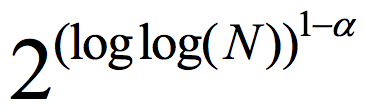for some constant <1.3

All of this dramatically changed last year. Cohen,4 and independently Chattopadhyay and Zuckerman,5 constructed K-Ramsey graphs with log K=poly loglog(N). Remarkably, Chattopadhyay and Zuckerman explicitly construct the stronger object of a two-source extractor. A function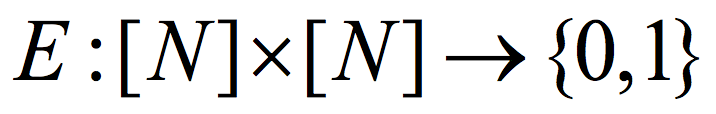is a (K,ϵ) 2-source extractor if for every two cardinality K subsets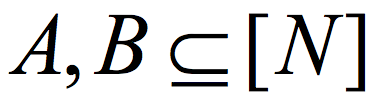, the distribution E(A,B) (obtained by picking uniformly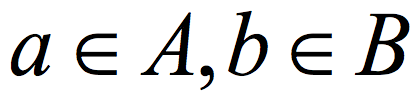and outputting E(a,b)) is ε close to uniform in the variational distance. Roughly speaking, the Ramsey graph problem is to find a (K, ε) 2-source extractor with any error ε= ε(K) smaller than 1, even allowing error that is exponentially close to 1. In contrast, The CZ construction gives a 2-source extractor with constant error. A 2-source extractor is a basic object of fundamental importance, and the previous best 2-source construction was Bourgain’s extractor, requiring log(K) =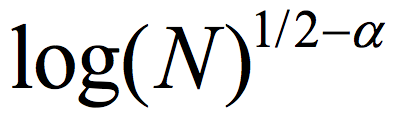for some small constant α>0. The CZ construction is an exponential improvement over that, requiring only log(K)=polyloglog(N).

How does the CZ construction work? Roughly speaking, the first step in the CZ construction is to encode a sample from one source (say) with a t non-malleable extractor. I do not want to formally define non-malleability here, but this essentially means that the bits of the encoded string are “almost” t-wise independent, in the sense that except for few “bad” bits, when we look at t bits, they are close to being uniform. The next step in the CZ construction is to use the sample from the second source (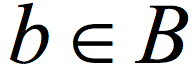) to sample a substring of the encoding of a. The sampling is done using an extractor and uses the known relationship between extractors and samplers.6 Finally, a deterministic function, conceptually similar to the Majority function, is applied on the bits of the substring.

The CZ construction achieves log K=polyloglog(N), where the non-explicit argument of Erdős shows log K=loglog(N)+1 is sufficient. The first bottleneck in the CZ construction, pointed out by Cohen and Schulman,7 is the use of extractors as samplers in the construction. In a recent work, Ben-Aroya, Doron and I showed how to solve this problem using samplers with multiplicative error.8 Furthermore, Dodis et al. showed such samplers are related to low entropy-gap condensers, and Yevgeniy gave a series of talks on such condensers and their applications, mainly in cryptography, during the Spring 2017 Simons Institute program on Pseudorandomness.9 With this, the currently best explicit construction has log K=loglog(N) polylogloglog(N). The extra polylogloglog(N) factor is because current explicit t-non-malleable constructions, even for a constant t, have a suboptimal dependence on ε.

Yet, in my opinion, the most pressing bottleneck is of a completely different nature. The CZ result efficiently constructs a two-source (K,ε) extractor for small values of K, but a large error ε. Specifically, the algorithm computing E(a,b) has running time poly(1/ε), and if we take explicitness to mean running time polynomial in the input length log(N), we can only hope for error which is 1/polylog(N). In contrast, a straightforward probabilistic method argument shows we can hope for an ε which is polynomially small in K. The currently best low-error constructions are Bourgain’s 2-source extractor requiring log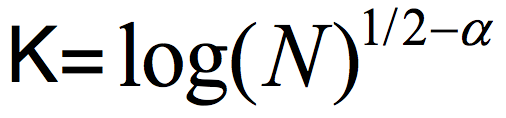and Raz’ extractor which allows one source to be (almost) arbitrarily weak, but requires the other source to have min-entropy rate above half (the entropy rate is the min-entropy divided by the length of the string). At the Simons Institute program on Pseudorandomness, we were wondering whether the CZ approach that allows both sources to be weak can be somehow modified to give a low-error construction?

# Research Vignette: Promise and Limitations of Generative Adversarial Nets (GANs)If we are asked to close our eyes and describe an imaginary beach scene, we can usually do so in great detail. Can a machine learn to do something analogous, namely, generate realistic and novel images different from those it has seen before? One expects that some day, machines will be creative and be able to generate even new essays, songs, etc., but for this article, let’s discuss only images. In machine learning, this goal of generating novel images has been formalized as follows. We hypothesize that realistic images are drawn from a probability distribution on the (vast) space of all possible images. Humans appear to be able to imagine novel samples from this vast distribution after having seen a reasonably small number of examples that arose in their personal experience. We would like machines to do the same: sample from the distribution of all realistic images. While this framing of the problem appears anticlimactic – reducing creativity/imagination to the more prosaic act of learning to generate samples from a vast distribution – it is nevertheless a powerful framework that already presents difficult computational hurdles. Many past approaches for solving this distribution learning problem used explicit statistical models, and tended to end in failure (or at best, tepid success) because real life data is just too complicated to capture using simple models.

This article is about Generative Adversarial Nets (GANs), a proposal by Goodfellow et al. in 20141 to solve this task by harnessing the power of large-scale deep learning (sometimes also called neural net training). Specifically, in the last 5-6 years, deep learning has become very successful at teaching machines to recognize familiar objects in images, in the sense that they can give labels to scenes such as street, tree, person, bicyclist, parked cars with precision approaching or exceeding that of humans. Perhaps this ability can lead naturally to a solution of the sampling problem?

# Research Vignette: Setting Posted Prices Under Uncertainty

Selfish Behaviour and Uncertainty
The author had the undeserved great fortune to be invited to two semesters at the Simons Institute for the Theory of Computing. The author had a truly wondrous time and is immensely grateful for the this fantastic opportunity. The opportunity to hear and interact with the amazing people attending was priceless. Thus, when asked to write a short Research Vignette,1 the author felt that it would be churlish to object strenuously. One can but hope that this is not yet another example of an error in judgment.2

Aspects of uncertainty have been studied in many disciplines, including philosophy, psychology, physics, the life sciences, economics and computer science, among others. In this Vignette, we address some aspects and models of uncertainty in statistics, computer science, and economics.

Temporal uncertainty occurs where the future or certain aspects of the future are unknown. Decisions made today (e.g., agreeing to write this article) may have unforseen consequences tomorrow (when the disastrous NYT critique is published). Optimal stopping theory,3 prophet inequality settings,4 secretary settings,5 and competitive analysis of online algorithms6 all deal with aspects of and models for temporal uncertainty. This vast body of work includes both Bayesian and worst-case models.

Another element of uncertainty arises when selfish agents interact. Clearly, it is useful to know how much a customer is willing to pay before trying to sell her something. Given that the techniques used by Torquemada are often condemned,7 one needs to be more subtle when considering the impact of private information.

One approach is to consider rational behavior and study resulting equilibria.8 Pricing equilibria (competitive equilibria) have been studied in many settings.9 Social choice theory10 and mechanism design11 seek to engineer algorithms so as to achieve certain desired goals. A wide variety of equilibrium notions have been defined, including dominant strategy, Bayes-Nash, and many others.

The computer science outlook is to quantify things – in this case, how good an outcome arises in equilibria when compared to optimal outcomes,12 and what mechanisms can be implemented in poly time.13

The setting of interest
Consider a model of online decision-making mechanisms in which selfish agents arrive sequentially, and the mechanism decides upon an outcome and payment for each arriving agent, where payments are used to align the incentives of the agent with that of the mechanism. There is temporal uncertainty because the preferences of the agents (their types) are unknown. The agent may have an opportunity to specify her type, but the decisions made by the mechanism might not be in the best interest of the agent. This may result in the agent strategically misrepresenting her preferences so as to achieve a better outcome for herself. A mechanism is truthful if it is always in the best interest of the agent to report her type truthfully.

For some time I have been obsessed with a specific class of truthful online mechanisms that take the form of dynamic posted prices.14 The assumption here is that the future is entirely unknown, and can be determined adversarially with no guarantees on future behavior. Dynamic pricing schemes are truthful online mechanisms that post prices for every possible outcome, before the next agent arrives. Then, the agent chooses the preferred outcome — minimizing the cost for the outcome plus the price tag associated with the outcome.

Online problems are either minimization problems (e.g., minimize the sum of costs) or maximization problems (e.g., maximize the number of agents served). Although optimal solutions to maximization/minimization objectives can be cast as the other, the competitive ratio is quite different in the two settings. Online algorithms have been devised with both maximization and minimization objectives. The technique of “classify and randomly select” often gives simple randomized algorithms for maximization objectives which also naturally translate into truthful mechanisms. In contrast, minimization objectives (e.g., k-server and makespan) require entirely different techniques. Converting online algorithms into mechanisms without performance degradation opens up an entire new class of problems for which incentive compatible mechanism design is applicable.

We note there are many other problems where posted prices play a major role, within widely differing models, information states, and various stochastic assumptions.15

A dynamic pricing scheme is inherently truthful, since prices are determined irrespective of the type of the next agent. Posted price mechanisms have many additional advantages over arbitrary truthful online mechanisms. In particular, such mechanisms are simple: agents need not trust or understand the logic underlying the truthful mechanism, agents are not required to reveal their type, and there is no need to verify that the agents follow the decision made by the truthful online mechanism.

A posted price mechanism is a truthful online algorithm, and as such, can perform no better than the best online algorithm. The main goal of this approach is to study the performance of dynamic posted price mechanisms (quantified by the competitive ratio measure) and compare them with the performance of the best online algorithm. One may think of this problem as analogous to one of the central questions in algorithmic mechanism design in offline settings: compare the performance of the best truthful mechanism (quantified by the approximation ratio measure) with the performance of the best non-truthful algorithm.

In a paper at EC 2017, Feldman, Roytman and I presented constant competitive dynamic pricing schemes for makespan minimization in job scheduling. Events represent jobs; the job type contains the job’s processing times on various machines. Agents seek to complete their job as soon as possible, and therefore prefer to be assigned to a machine whose load (including the new job) is minimized.16 One can consider so-called related machines (where machines have speeds, and jobs have some quantity of work to be done) and unrelated machines (where the time associated with a job arbitrarily depends on the machine). Previous online algorithms for the problem17 are not truthful — in that a job may misrepresent its size so as to get a preferential assignment to a machine.

An online truthful mechanism for this setting determines an allocation and payment for each arriving agent upon arrival. That is, upon the arrival of a job, based on the job’s processing times, the mechanism assigns the job to some machine and determines the payment the agent should make. The cost of an agent is the sum of the machine’s load (including her own processing time) and the payment. Each agent seeks to minimize her cost.

A dynamic posted price mechanism for this setting sets prices on each machine, before the next agent arrives (prices may change over time). The next agent to arrive seeks to minimize her cost, i.e., the load on the chosen machine (including her own load) plus the posted price on the machine. The agent breaks ties arbitrarily.

An example of dynamic pricing: Makespan minimization, related machines
We now motivate how dynamic pricing is useful, via a small example. We do so by comparing the schedule produced without any pricing to the schedule produced via a dynamic pricing scheme. Schedules obtained without pricing are equivalent to schedules produced by the greedy algorithm that assigns each job j to a machine i that minimizes ℓi(j − 1) + (the load on machine i prior to the arrival of job j, plus the additional time required for job j itself).

Figure 1: Related machines: the optimal makespan, the greedy algorithm, and dynamic pricing. The algorithm for setting dynamic prices is missing.

In our example (given as Figure 1) there are m = 3 machines, with speeds s1 = , s2 = (1 + ϵ), and s3 = 1 + 2ϵ; and n = 3 jobs, with sizes p1 = (1 + ϵ), p2 = , and p3 = 1 + 2ϵ. The left, middle, and right columns show the optimal assignment, the greedy assignment, and the assignment obtained by our dynamic pricing scheme, respectively. In the middle and right columns, the arrival order is from bottom to top.

Optimal makespan: The optimal makespan is L = 1, achieved by assigning job 1 to machine 2, job 2 to machine 1, and job 3 to machine 3.

Greedy: The greedy algorithm assigns job 1 to machine 3, since the machine i that minimizes is the fastest machine (initially, all loads are 0). Job 2 is also assigned to machine 3, since ℓ3(1) + = < = < (for sufficiently small ϵ > 0). Lastly, job 3 is also assigned to machine 3, since ℓ3(2) + = < = < . Hence, the greedy algorithm assigns all jobs to machine 3, resulting in a makespan of ≈ 2.

Pricing scheme: Our dynamic pricing scheme sets prices before the arrival of each job, which are independent of the type of the incoming job. We omit the explanation as to how to set these prices.18 The important aspect is that these prices are set before knowing what the next job will be. The performance guarantee must hold irrespective of the future. Let cij be the cost of job j on machine i; this is the sum of the completion time and the additional posted price currently on the machine.

Job 1 chooses machine 1, since c11 = + π11 = 1 + ϵ < 1 + π21 = + π21 = c21 < + 1 + π21 + π31 = c31.

Prior to the arrival of job 2, the dynamic pricing scheme sets new prices (see Figure 1), and job 2 chooses machine 2, since c22 = + π22 < + 1 + ϵ + = ℓ1(1) + = c12 < + 2 ≈ + π32 = c32.

Finally, prior to the arrival of job 3, the dynamic pricing scheme sets yet new prices and  job 3 chooses machine 3, since c33 = + π33, while c13 = ℓ1(2) + = 1 + ϵ + 2p3 ≈ 3 and c23 = ℓ2(2) + + π23 = + + π23 ≈ 3.

Since machine 1 has the highest load, the schedule produced by our dynamic pricing scheme achieves a makespan of ℓ1(3) = 1 + ϵ. This example can be extended to show that greedy can be as bad as Ω(log m)-competitive, while in contrast our dynamic pricing scheme is O(1)-competitive.

During a talk in the Fall 2017 Simons Institute program on Algorithms and Uncertainty, I proposed that one study dynamic pricing schemes for flow time; and indeed, one definite outcome of this semester is a paper by Im, Moseley, Pruhs and Stein that comes up with a constant competitive dynamic pricing scheme to minimize the maximum flow time (the maximum time an agent is in the system). This improves on the previous online algorithm for the problem in terms of the competitive ratio, and has the added advantage of being truthful and simple.

Online algorithms, online mechanisms, and dynamic pricing
One critical question is the following: where do the boundaries lie between online algorithms, online mechanisms (not dynamic pricing) and dynamic pricing? In our EC paper, we show that there exist problems – makespan minimization on unrelated machines, where the pair (job, machine) determines the processing time – where good online mechanisms exist but there are no good dyanmic pricing schemes.

The connection between online mechanisms and dynamic pricing schemes is not entirely trivial.19 Dynamic pricing schemes are obviously truthful mechanisms, but converting an arbitrary online mechanism into a dynamic pricing scheme may not be possible.

We can show that online mechanisms with certain performance guarantees that also have properties a to c below can be converted into dynamic pricing schemes with the same guarantee: a) the online mechanism must be prompt (where the payments are determined immediately); b) ties in agent utilities do not arise, or can be resolved arbitrarily without harming performance guarantees — this is not an issue in the mechanism-design setting because the mechanism may decide how to break ties, but it is an issue with pricing schemes; and c) the mechanism does not require “voluntary revelation” of agent types – in pricing schemes, the only information available to the dynamic pricing scheme is what the previous agents actually chose; pricing schemes are not direct revelation schemes.

Afterword
It has been a pleasure discussing these and other problems with researchers visiting the Simons Institute, and at my home site, Tel Aviv University. I am grateful for discussions with and gracious instruction from Nikhil Bansal, Avrim Blum, Alon Eden, Michal Feldman, Anupam Gupta, Ilia Gorlick, Haim Kaplan, Anna Karlin, Dick Karp, Thomas Kesselheim, Bobby Kleinberg, Elias Koutsoupias, Stefano Leonardi, Christos Papadimitriou, Kirk Pruhs, Tim Roughgarden, and Matt Weinberg. In various combinations, we have tried to attack many other online problems via dynamic pricing. Many of these are wide open; some (minor) progress has been made on others. Many an idea arose in the numerous talks and discussions at the Simons Institute.

### Notes

1Merriam-Webster online dictionary: 2a) a short descriptive literary sketch; 2b) a brief incident or scene (as in a play or movie).

2According to Aristole (Poetics, 4rd century BCE), an ideal tragedy is caused by error in judgment by the protagonist, and not by unavoidable error.

3Wald (1945), Arrow, Blackwell and Girshick (1948); Snell (1952).

4Cayley (1875); Krengel, Sucheston and Garling (1977).

5Kepler (1613); Martin Gardner (1960); Gibert and Mosteler (1966).

6Sleator and Tarjan (1985).

7Recent political developments in North America notwithstanding.

8von Neumann (1928).

9Cournot (1838); Walras (1874); Arrow and Debreu (1954).

10Condorcet (1785); Arrow (1951); Gibbard and Satterthwaite (1973,1975).

13Nisan and Ronen (1999).

14Fiat, Mansour and Nadav (2008) — Packet routing; Cohen-Addad, Eden, Fiat and Jeż (2015) — Task systems, k-server, and metrical matching; Feldman, Fiat and Roytman (2017) — Makespan minimization; Im, Moseley, Pruhs and Stein (2017), the latter two discussed herein.

15E.g., Myerson’s virtual values, prophet inequalities, secretary problems, combinatorial markets – Feldman, Gavin and Lucier (2013, 2015 and 2016); Envy-free pricings – Guruswami, Hartline, Karlin, Kempe, Kenyon and McSherry (2005); and many others.

16In this interpretation, “load” is the time required by the server to deal with all current jobs in the server queue, and jobs are processed in a first-in, first-out manner, i.e., jobs enter a server queue.

17Awerbuch, Azar, Fiat, Plotkin and Waarts (1993).

18EC17 and on the archive.

19Many thanks to Moshe Babaioff, Liad Blumrosen, Yannai A. Gonczarowski and Noam Nisan for discussions helpful in clarifying this point.

# Research Vignette: Entangled Solutions to Logic Puzzles

The Fall 2016 Simons Institute program on Logical Structures and Computation focused on four themes: a) finite and algorithmic model theory; b) logic and probability; c) logic and quantum mechanics; and d) logic and databases. In this Research Vignette, I want to highlight one of the outcomes of this fruitful program in the form of an emerging direction of research that, quite surprisingly, touches on all four themes at once.

Two-player refereed games
A game is played by Alice and Bob, who confront a verifier. After an initial gathering to agree on a common strategy, Alice and Bob will not be allowed to communicate during the game. The game starts with the verifier privately choosing a pair of queries (p,q) ∈ P × Q according to some probability distribution π known to everyone; the verifier sends p to Alice and q to Bob. Alice and Bob reply with a pair of answers (r,s) ∈ R × S according to their previously agreed strategy; Alice replies r and Bob replies s. Upon receipt of the answers, the verifier accepts or rejects depending on whether a predicate V (p,q,r,s) also known to everyone holds or not. Alice’s and Bob’s goal is to maximize the probability of winning, i.e., making the verifier accept. Let this maximum be called the value of the game.

How could we model the value of this game? One perfectly natural approach would be to model it as a constraint-optimization problem. The possible queries are the variables, the possible answers are the values for these variables, and the verifier’s predicate V (p,q,r,s) defines the constraints, weighted by π(p,q). The optimal strategy would then be an assignment of values to variables that maximizes the total weight of satisfied constraints.

However, this model does not seem to capture all the different ways Alice and Bob could interact in their initial gathering. For example, Alice and Bob could have agreed to use the outcomes of a number of coin-flips, which they could have recorded in their respective memory sticks, to determine their answers during the game. As long as they don’t communicate during the game, this form of interaction is allowed! A better model for this situation would then seem to be that Alice and Bob choose functions of the form A : P × T R and B : Q × T S, where T denotes the set of potential coin-flip results, which has an associated probability distribution σ. Their goal would now be to maximize the average weight:

It turns out that in this particular case, there is no difference in the optimal value of the two models; but one could easily imagine that if we missed this one, we could well be missing other ways that Alice and Bob could interact in defining their strategy. And indeed, we are missing some such forms of interaction.

Suppose that Alice and Bob, when they design their strategy, agree to prepare a pair of elementary particles in a suitable entangled state, in the way they were taught was possible in their quantum mechanics class. When they separate, Alice takes one of the particles with her and Bob takes the other. When they play, Alice and Bob perform certain measurements on their respective particles depending on the query each one gets, and then they use the outcomes to determine their answers. Notice that Alice and Bob still stay separated from each other; they just perform local measurements on their local particles! The question is: could the fact that the particles were entangled in the past give Alice and Bob a strategy that has greater probability of winning?

Quantum vs. hidden-variable theories
The peculiar properties of quantum entanglement have fascinated physicists, mathematicians, logicians, and philosophers since the 1920’s. It is well-known that such giants as Einstein were initially reluctant to accept the uncertainty principle of quantum mechanics in its full strength. Others, such as von Neumann, were profoundly inspired by the topic to develop their deepest work. In more recent times, theoretical computer scientists have brought forth a computational and information-theoretic perspective that, hopefully, sheds some more light. The origins of this approach go back to the famous 1964 article of J.S. Bell, “On the Einstein-Podolsky-Rosen Paradox.”

Following Bell, let’s consider a Gedankenexperiment. Before the experiment starts, a large number N of pairs of particles of so-called spinare independently prepared in an entangled state, the singlet state. The experiment starts when Alice and Bob travel to distant planets with one particle each from each pair. Once in place, they measure the spins of their particles along different axes. Alice uses axis a and Bob uses axis b, where a and b are 3-dimensional unit vectors chosen by the verifier. As they do their measurements, they collect outcomes r1,,rN ∈{+1,−1} for Alice, and s1,,sN ∈{+1,−1} for Bob, which they send back to the verifier. At this point Bell asked: what are we to expect for the value of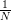i=1Ns iri? In other words, if the verifier counts the number of times that ri = si and subtracts the number of times that ri ≠ si, what are we to expect?

Bell computed what quantum theory predicts for these questions, and he concluded that one is to expect that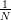i=1Nr isi approaches − cos θ as N grows, where θ ∈  is the angle between the axis vectors a and b. Put differently, if we ask the verifier to accept any single pair of data (ri,si) only if ri = si, one is to expect that the verifier would accept with probability approachingcos θ. The point of Bell’s study was to contrast this calculation with what would be expected from a local hidden-variable theory of the type Einstein and his co-authors had hoped would explain the uncertainty in the experiment. In such a model, Bell argued, the statistics ofi=1Nr isi would have to take the form of an average ∑ tT σ(t)i=1Nr i(t)si(t), and he went on to prove that in such a case it would have to approach the quantity −1 +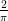θ. In other words, a verifier that accepts only if ri = si would have to accept with probability approaching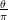. The discrepancy between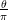and the earliercos θ is particularly noticeable at θ ≈ 133.56 where the ratio is minimized and is as small as 0.878567.

The analysis in Bell’s Theorem can be turned into a game in which entangled strategies give higher probability of winning than unentangled ones. The question arises, however, of whether this difference can be witnessed only statistically, i.e., quantitatively, or if it manifests itself in more absolute terms, i.e., qualitatively. In other words, could there be a game in which Alice and Bob can make the verifier accept with certainty if they use entanglement but not if they don’t? Such questions had also been considered by physicists, including Bell himself, with the conclusion that, also at this level, entangled strategies are superior to unentangled ones. This type of refinement of the sense in which entanglement is more powerful, which led Mermin to coin Kell-Kochen-Specker type theorems in his beautiful paper, “Hidden variables and the two theorems of John Bell,” ties better to the problems of interest to logicians, and will take our attention for the rest of this Vignette.

Quantum relaxations of constraint satisfaction problems
Take an instance of the constraint satisfaction problem with Boolean variables X1,,Xn ranging over {+1,−1}, and constraints given by, say, polynomial equations of the form P(Xi1,,Xik) = 0. There is a well-known game that models this problem: the verifier chooses both a constraint and a variable that appears in this constraint, sends the constraint to Alice and the variable to Bob, and expects an assignment that satisfies the constraint from Alice, and an assignment that agrees with Alice’s on the pointed variable from Bob. It is easy to see that the solutions to the constraint-satisfaction instance give rise to (unentangled) strategies that win with certainty in the game, and vice-versa. What about entangled strategies? What do they correspond to at the level of the constraint-satisfaction instance? In their ICALP 2014 paper, theoretical computer scientists Richard Cleve and Rajat Mittal addressed this question and, to answer it, introduced the following quantum relaxation of the constraint-satisfaction problem:

Let the variables X1,,Xn range over Hermitian d-dimensional matrices in ℂd×d for some arbitrary but unspecified dimension d ≥ 1. Impose the conditions that Xi2 = I, where I is the identity matrix, and that the matrix product on Xi and Xj commutes whenever Xi and Xj appear together in at least one of the given polynomial equations. Note that the case d = 1 is no relaxation at all since the 1-dimensional matrices are just scalars, whose product obviously commutes, and the equations Xi2 = I translate to X i ∈{+1,−1}. Cleve and Mittal proved that entangled winning strategies in the game give rise to solutions to this relaxed version of the problem, and vice-versa.

Now we can ask the type of questions that Bell asked. Could there be a system of polynomial equations that is quantum-satisfiable in the sense above but not classically satisfiable? Some well-known such examples come from the work of the physicists in their proofs of the Bell-Kochen-Specker Theorems; the best known is the Mermin-Peres Magic Square made of six polynomial equations (the three rows and the three columns in the square below) over nine variables:

Seen as a logic puzzle of the kind that one used to find in newspapers, this appears to have no solution: all variables appear exactly twice so the left-hand sides of the equations multiplied together give 1, while the right-hand sides give −1. In contrast, the system is quantum-satisfiable by means of a 4-dimensional solution made of suitable Kronecker products of the classical Pauli matrices (Fig 3. in Mermin’s paper — see Notes for further reading, below).

Emerging directions
In his series of lectures in the Logic and Computation Boot Camp that took place at the begining of the program, Samson Abramsky gave an introduction to the topic of Bell-type results from a logician’s viewpoint. A recurrent theme within the lectures was the extent to which local constraints on a system, such as the outcomes of physical measurements, may or may not influence its global properties. Put this generally, this is indeed a common theme of most subareas of logic in computer science. It appeared in Panangaden’s lectures on the analysis of probabilistic systems while constructing continuous probability spaces that meet the local constraints that arise from conditioning. It also appeared in Kolaitis’ lectures on database theory while discussing how the local structure of a conjunctive query can influence the size of its output and the computational complexity of computing it. And it appeared in Dawar’s course on finite and algorithmic model theory as the fundamental question of whether the local structure of a finite model could determine it up to isomorphism. I will close this Vignette with a discussion on a research question that was raised at the program, which links this last topic with Bell-Kochen-Specker-type theorems, and which was successfully answered in a team-effort during the program.

The work of Cleve and Mittal referred to above is the continuation of a recent trend that takes classical combinatorial problems and relaxes them into quantum analogues: quantum chromatic numbers, quantum homomorphisms, quantum Lovász theta, etc. Let us take quantum graph isomorphism. We are given two graphs G and H. The verifier chooses two vertices from either graph and sends them to Alice and Bob, one vertex each. If Alice gets a vertex u from G, she must reply with a vertex v from H, and vice-versa. If Bob gets a vertex u′ from G, he must reply with a vertex v′ from H, and vice-versa. The verifier will accept if and only if the two pointed vertices from G satisfy the same equalities and edge relationships as the two pointed vertices from H; i.e., he will accept precisely when u = u′ if and only if v = v′, and u Gu′ if and only if v Hv′. It is not hard to see that Alice and Bob have an unentangled strategy that wins this game with certainty if and only if G and H are isomorphic. Are there pairs of graphs on which Alice and Bob win with entanglement but not without?

The graph isomorphism problem is of course of fundamental importance in theoretical computer science. For one thing, it is one of the few problems in the complexity class NP that is not known to be polynomial-time solvable or NP-complete. Thanks to the recent breakthough of Babai, we now know that the problem is solvable in quasipolynomial time, and thanks to the earlier work in probabilistic proof systems, we already had strong evidence that the problem cannot be NP-complete. These two facts could explain why it has always been so hard to find hard-to-solve instances of the graph isomorphism problem. To put it graphically, if you give me a reasonably smart heuristic to solve the graph isomorphism problem, chances are that I will have a hard time finding an example on which your heuristic fails. For this very same reason, finding two graphs on which Alice and Bob can win the isomorphism game with entanglement but not without does not look like a very easy question.

It turns out that similar questions had been asked previously in descriptive complexity theory in the 1980’s. The fundamental problem of that area, which has its origins in the theory of relational databases, is whether there is a logic that is able to express all and only the isomorphism-invariant graph properties that can be decided in polynomial time. In the 1980’s some candidate logics were put forward, until one was found to resist all attempts at a counterexample. This was the logic now called Fixed-Point Logic with Counting (FPC). Eventually, in a breakthrough that became known as the CFI-construction, J. Y. Cai, M. Fürer and N. Immerman found a counterexample: they proved that the isomorphism problem for graphs with color-classes of bounded size, which is a special case that is solvable in polynomial time, cannot be expressed in FPC.

Besides its original purpose, the CFI-construction became also the canonical “hard-to-solve” instance for combinatorial heuristics for the graph isomorphism problem. For one thing, it was the first construction to show that there exist pairs of non-isomorphic graphs for which the classical vertex-refinement heuristic, when extended to tuple-refinement in the straightforward way, would need to use tuples of unbounded length before it detects that the graphs are not isomorphic. Could the same construction be used to solve our problem of finding non-isomorphic graphs that are nonetheless quantum isomorphic? One difficulty in going this way is that nobody knows of a systematic method to decide if two given graphs are quantum isomorphic; as far as we know, the problem could be even undecidable. For this reason, it would have been more natural to start by constructing two graphs that are quantum isomorphic by design, and only then show that they are not classically isomorphic. But CFI was already there, and we had to give it a try. So we tried it, and… it worked!

The key to finding the solution was the previously noted fact that, in the context of the logical and universal-algebraic approaches to the Feder-Vardi Dichotomy Conjecture for constraint satisfaction problems, the CFI-construction can be interpreted as a system of parity equations in which each variable appears in exactly two equations. Coincidentally (or not), these are precisely the type of systems of equations that underly the Mermin-Peres Magic Square and similar constructions. From there, a few calculations sufficed to realize that Mermin’s solution to the magic square could be reused, and the problem was solved.

Bell’s paper appears in Physics 1, 1964: 195-200. The ratio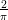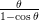in our exposition of Bell’s analysis is exactly the same as Goemans-Williamson’s approximation ratio for MAX-CUT and is related to Grothendieck’s constant; this is hardly a coincidence. See for example the paper by Regev and Vidick, “Quantum XOR Games,” ACM TOCT, 7(4), 2015, n. 15, and references therein. For the Bell-Kochen-Specker Theorem and the magic square see Mermin’s Rev. Mod. Phy. 65(3), 1993. Cleve and Mittal’s paper appeared in the Proceedings of ICALP 2014 and also QIP 2014 and arXiv:1209.2729 [quant-ph]. The Cai-Fürer-Immerman paper appears in Combinatorica 12(4), 1992: 389-410, with an earlier version in FOCS 1989. The new results on quantum graph isomorphism appear in Atserias, Mančinska, Roberson, Šámal, Severini, and Varvitsiotis, “Quantum and non-signalling graph isomorphisms,” arXiv:1611.09837 [quant-ph], with a shorter version in ICALP 2017. Two other recent papers that report work along these lines are Atserias, Kolaitis and Severini, “Generalized Satisfiability Problems via Operator Assignments,” arXiv:1704.01736 [cs.LO]; and Abramsky, Dawar and Wang, “The pebbling comonad in finite model theory,” arXiv:1704.05124 [cs.LO]. This last will appear in shorter form in LICS 2017.

# Research Vignette: Network Biology Meets Cancer Genomics

by Teresa Przytycka and Roded Sharan

The Spring 2016 Simons Institute program on Algorithmic Challenges in Genomics focused on three main research areas: Computational Cancer Biology, Regulatory Genomics and Epigenomics, and Network Biology. The different tracks allowed us, the long-term visitors, to better understand the relationships among the different areas and come up with ideas on how they can be integrated to gain further insights into biology. Here, we would like to tell the story of one such integration attempt that was initiated during the Simons program, where ideas from network biology led to new formulations and novel findings in cancer genomics.

A fundamental concept in cancer genomics is that of a disease module that contains functionally related genes that jointly drive the disease. The discovery of such gene sets can reveal the molecular mechanisms that underlie the disease and suggest potential therapeutic targets. It is generally assumed that cancer progresses through stages, starting from a precancerous lesion caused, for example, by DNA damage. If such damage cannot be properly handled by the cell repair mechanisms, for example when such mechanisms are compromised by mutations, an early stage of cancer emerges. In this state, the organism still relies on cellular mechanisms such as apoptosis, cell cycle arrest, or senescence, to guard against uncontrolled cell growth and proliferation. However, once those barriers are compromised, say by further mutations, the disease progresses. While the progression seems sequential, steered by driver mutations that provide a growth advantage to the evolving tumor cells, the actual process is more complex and not fully understood. To shed light on the progression process, we need not only to be able to identify disease modules, but also to understand the relationships among them.

A common approach to detecting cancer modules is to look for sets of genes that are mutually exclusive in the mutations they harbor, across a cohort of patients. The underlying assumption is that the module can be disrupted by a mutation in any of its member genes, thereby driving the disease progression, and additional mutations do not provide an advantage to the disease. As driver mutations are selected for during cancer evolution, the module’s genes often exhibit mutual exclusivity mutation patterns across different patients. Other inter-gene relations such as physical interactions, co-expression, functional similarity, and more, can inform the discovery of cancer modules. Thanks to public projects, such as The Cancer Genome Atlas, researchers can now access genomic data of thousands of patients across tens of cancer types. Such valuable data sources enable the systematic search of disease modules using different computational and statistical approaches .

While several research projects have tried to infer disease modules from mutation data, most have focused on mutual exclusivity relations. Only a few projects have considered co-occurrence relations, the integration of other types of data, or the modeling of inter-module relations. These more complex scenarios, however, are very common in network biology, where multiple relations can be modeled by multiple edge types in a gene network. The discovery of interesting edge-patterns can then be cast as a clustering problem where one aims to maximize within-cluster associations as well as between-cluster relations. It thus seemed natural to try and apply such general clustering formulations in the cancer genomics domain.

Multiple relations can exist within and between genes of true cancer modules. We already mentioned mutual exclusivity within modules (Figure, Panel A), but it can also be present between modules, representing different cancer subtypes or different cancer progression pathways (Figure, Panel B). Similarly, mutations can co-occur within modules, representing some shared mutagenic process, or between modules, representing a synergistic relation, where two alternative pathways need to be disrupted for the disease to occur (Figure, Panel C). Finally, known gene interactions or functional associations are typically within modules, representing their shared role in some molecular pathway driving the disease.

To capture all these different relations, Phuong Dao, Yoo-Ah Kim, Damian Wojtowicz, Sanna Madan and I modeled them within a general clustering framework that scores a given collection of modules by their within- and between-edges. The resulting clustering problem is reminiscent of cluster editing, also known as correlation clustering, where one wishes to minimally edit the edge set of a given graph so that it is transformed into a collection of disjoint cliques. While the problem is known to be NP-hard , it admits a practical and exact solution via integer linear programming . Motivated by the success of the latter approach, we formulated the within-between module detection problem using integer linear programming. By applying a commercial solver (Cplex), we could solve the problem to optimality on current data sets, consisting of hundreds of mutated genes and thousands of relations across hundreds of patients, in less than an hour. The integrated network view of these relations made it possible to systematically test the contribution of the different types of relations, both within and between modules, to module discovery.

In an application to real data from The Cancer Genome Atlas project, we were able to form comprehensive maps of the modules underlying different cancers and gain novel insights about the roles of different genes in driving the disease. As an example, when maximizing the mutual exclusivity of mutations within modules and the co-occurrence of mutations between modules, we uncovered pairs of modules that work in a synergistic fashion, where the disruption of both is required for the disease to occur (Figure, Panel C). Overall, our general strategy that accounts for a number of complementary relations proved to be a valuable tool in dissecting the mutational landscape of cancer to infer molecular mechanisms that drive the disease.